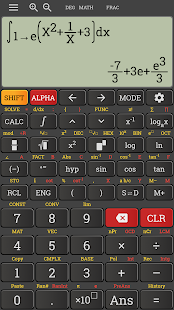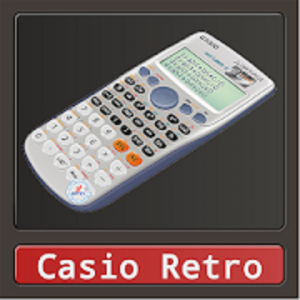# Natural mathematics display calculator

This calculator support full math functions, such as derivative, integral, equation solving. This calculator supports some features of calculator fx 4500, fx 260, fx 500, JX570ms, fx-82AU PLUS II, fx-82 or 991ms, 570vnplus, 82ms, 82es, fx570esplus, 570es, 82 eu, TI-30XB, 991ex, fx500, fx 4500pa, 991es plus, 100AU, sharp el738, fx 580 vnx/ fx 580 vn

The Natural Display shows mathematical expressions like roots and fractions as they appear in your textbook, and this increases comprehension because results are easier to understand

Memory
* Repeat function
* Variable memory: 9
* Number of storable programs: Calc / Solv button

Basic mathematics
* Functions
* Scientific constants
* Unit conversions
* Fraction calculations
* Coordinate conversion Pol
* Trigonometric functions sin/cos/tan/sin-1/cos-1/tan-1
* Hyperbolic functions sinh/cosh/tanh/sinh-1/cosh-1/tanh-1
* Exponent, log, In, 10x, ex
* Mathematical functions
* Calculations based on n (hexadecimal/decimal/binary/octal)
* Logical operators (AND/OR/etc.)
* Calculations in the sexagesimal system
* Percentage calculations
* Remainder function
* Technical notation ENG/ENG
* Calculation of complex numbers
* Random number generator
* Random integers
* Calculation of the minimum or maximum of a quadratic function
* GCD/LCM function
* Base-N calculations
* Value table
* Recurring decimal fractions

Differential and integral calculus
* Numerical integral calculus
* Numerical differential calculus

What’s New
– Add math formulas to favorite
– 1000+ Math formulas
– Fixed some bugs and minor improvements

Screenshots

•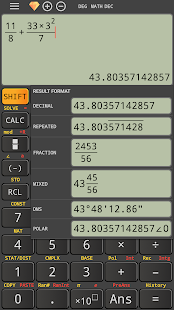••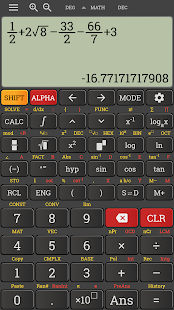••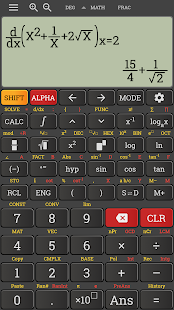•